# Common Core: High School - Geometry : Represent and Compare Transformations in the Plane: CCSS.Math.Content.HSG-CO.A.2

## Example Questions

← Previous 1

### Example Question #1 : Represent And Compare Transformations In The Plane: Ccss.Math.Content.Hsg Co.A.2

A basketball player is able to perfectly spin a basketball on his index finger. While the basketball is spinning, what mathematical expression can be concluded about it?

The basketball is not a transformation.

None of the other answer choices are correct.

Explanation:

This question is applying mathematical and more specifically, geometric definitions to describe a real life situation. Geometric definitions usually correspond to objects in the 2 dimensional plane or 3 dimensional surface. Since the basket ball is the object in question, this problem is dealing with a the 3 dimensional space.

From here, recall what is given

"A basketball player is able to perfectly spin a basketball on his index finger."

The index finger in this particular situation is acting as an axis for the basketball. Since the basketball is spinning and is maintaining its shape and size, this is considered a "rotation" in geometry.

### Example Question #1 : Represent And Compare Transformations In The Plane: Ccss.Math.Content.Hsg Co.A.2

A trolley car is attached to metal ground rails that go from the most southern block in a city to the most northern block of that same city. If the trolley starts at the south end and moves all the way to the northern end, what type of geometric expression does this situation define?

Translation

Transformation

Rotation

Both translation and transformation.

Reflection

Both translation and transformation.

Explanation:

To answer this question first recall the different geometric definitions that correspond to the listed possible answer choices.

Translation: To move an object from its original position a certain distance without changing the object in any other way.

Transformation: Refers to any of the four changes that can be done to an object geographically. Transformations include, reflections, translations, rotations, and resizing the object.

Reflection: To flip the orientation of an object over a specific line or function.

Rotation: To rotate an object either clockwise or counter clockwise around a center point.

Looking at the given situation,

"A trolley car is attached to metal ground rails that go from the most southern block in a city to the most northern block of that same city. If the trolley starts at the south end and moves all the way to the northern end, what type of geometric expression does this situation define?"

it can be seen that the trolley is not changing shape, it is not rotating nor reflecting. It is however, moving a certain distance from its starting position thus the definition of a translation and a transformation.

### Example Question #3 : Represent And Compare Transformations In The Plane: Ccss.Math.Content.Hsg Co.A.2

Dilation is a type of __________.

Rotation

Translation

Reflection

Transformation

Transformation

Explanation:

To answer this question first recall the different geometric definitions.

Translation: To move an object from its original position a certain distance without changing the object in any other way.

Transformation: Refers to any of the four changes that can be done to an object geographically. Transformations include, reflections, translations, rotations, and resizing the object.

Reflection: To flip the orientation of an object over a specific line or function.

Rotation: To rotate an object either clockwise or counter clockwise around a center point.

Since dilation is when an object changes size, that means dilation is a type of transformation.

### Example Question #11 : High School: Geometry

State the transformation of the red rectangle to the black rectangle.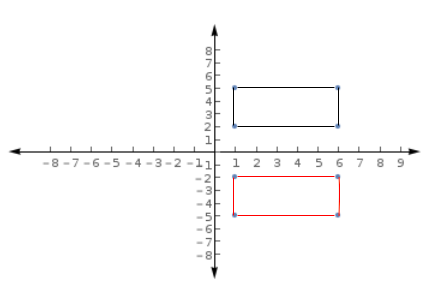Translation and Rotation

Rotation

Translation

Reflection and Translation

Reflection and Translation

Explanation:

To answer this question first recall the different geometric definitions that correspond to the listed possible answer choices.

Translation: To move an object from its original position a certain distance without changing the object in any other way.

Transformation: Refers to any of the four changes that can be done to an object geographically. Transformations include, reflections, translations, rotations, and resizing the object.

Reflection: To flip the orientation of an object over a specific line or function.

Rotation: To rotate an object either clockwise or counter clockwise around a center point.

Now, examine the given image and choose the appropriate answer.Looking at the red rectangle it is seen that the coordinate points of the vertices are: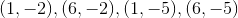The coordinate points of the vertices for the black triangle are: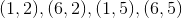Therefore, the red rectangle is being reflected over theaxis or it is being slide up four units. Thus, the correct answer is both a reflection and a translation.

### Example Question #1 : Represent And Compare Transformations In The Plane: Ccss.Math.Content.Hsg Co.A.2

Which of the following describes the change in the red polygon to the black polygon?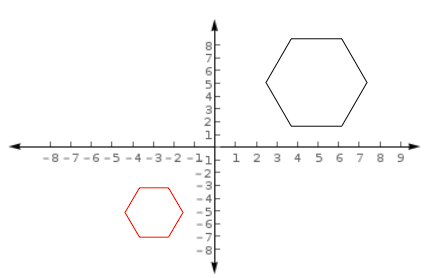Reflection

Rotation

Transformation and Translation

Translation and Rotation

Reflection and Translation

Transformation and Translation

Explanation:

To answer this question first recall the different geometric definitions that correspond to the listed possible answer choices.

Translation: To move an object from its original position a certain distance without changing the object in any other way.

Transformation: Refers to any of the four changes that can be done to an object geographically. Transformations include, reflections, translations, rotations, and resizing the object.

Reflection: To flip the orientation of an object over a specific line or function.

Rotation: To rotate an object either clockwise or counter clockwise around a center point.

Now, examine the given image and choose the appropriate answer.First, it is clear to see that the black polygon is larger than the red polygon. Therefore, a transformation has occurred since the original image has been resized. Also, the black polygon is in quadrant one while the red polygon is in quadrant three, therefore a slide or translation has occurred.

"Transformation and Translation"

### Example Question #12 : Congruence

Which of the following describes the change in the red circle to the black circle?

Assume the center of the circles are the same.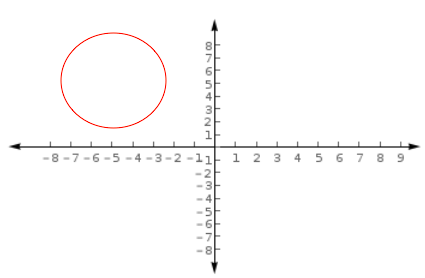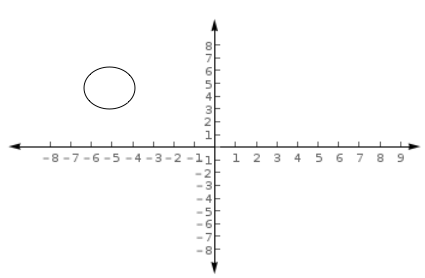Translation and Rotation

Rotation

Translation

Reflection

Transformation

Transformation

Explanation:

To answer this question first recall the different geometric definitions that correspond to the listed possible answer choices.

Translation: To move an object from its original position a certain distance without changing the object in any other way.

Transformation: Refers to any of the four changes that can be done to an object geographically. Transformations include, reflections, translations, rotations, and resizing the object.

Reflection: To flip the orientation of an object over a specific line or function.

Rotation: To rotate an object either clockwise or counter clockwise around a center point.

Now, examine the given image and choose the appropriate answer.First, it is clear to see that the black circle is smaller than the red circle. Therefore, a transformation has occurred since the original image has been resized. Since it is stated that the centers of the circles are the same it can be concluded that only a resizing has occurred.

"Transformation"

### Example Question #7 : Represent And Compare Transformations In The Plane: Ccss.Math.Content.Hsg Co.A.2

Determine whether the statement is true or false:

When a person's palm is faced down on a desk and that person then flips their hand over, their palm can be described as a reflection.

False

True

False

Explanation:

To answer this question first recall the different geometric definitions that correspond to the listed possible answer choices.

Translation: To move an object from its original position a certain distance without changing the object in any other way.

Transformation: Refers to any of the four changes that can be done to an object geographically. Transformations include, reflections, translations, rotations, and resizing the object.

Reflection: To flip the orientation of an object over a specific line or function.

Rotation: To rotate an object either clockwise or counter clockwise around a center point.

Now look at the specific event in this problem.

"When a person's palm is faced down on a desk and that person then flips their hand over, their palm can be described as a reflection."

Even though reflection is known as the "flipping" of an object it does not describe this situation because the palm of someones hand has different characteristics than the top of the hand therefore, the statement in this particular problem in false.

### Example Question #11 : Congruence

Which one of the following best describes a rotation?

Slide

Flip

Turn

Extend

Turn

Explanation:

To answer this question first recall the different geometric definitions.

Translation: To move an object from its original position a certain distance without changing the object in any other way.

Transformation: Refers to any of the four changes that can be done to an object geographically. Transformations include, reflections, translations, rotations, and resizing the object.

Reflection: To flip the orientation of an object over a specific line or function.

Rotation: To rotate or turn an object either clockwise or counter clockwise around a center point.

Therefore, the English term that best represents rotation is "turn".

### Example Question #21 : High School: Geometry

Which one of the following best describes a reflection?

Extend

Flip

Shrink

Slide

Flip

Explanation:

To answer this question first recall the different geometric definitions.

Translation: To move an object from its original position a certain distance without changing the object in any other way.

Transformation: Refers to any of the four changes that can be done to an object geographically. Transformations include, reflections, translations, rotations, and resizing the object.

Reflection: To flip the orientation of an object over a specific line or function.

Rotation: To rotate an object either clockwise or counter clockwise around a center point.

Therefore, the English term that best represents reflection is "flip".

### Example Question #21 : Congruence

Which one of the following best describes a translation?

Flip

Extend

Shrink

Slide

Turn

Slide

Explanation:

To answer this question first recall the different geometric definitions.

Translation: To move an object from its original position a certain distance without changing the object in any other way.

Transformation: Refers to any of the four changes that can be done to an object geographically. Transformations include, reflections, translations, rotations, and resizing the object.

Reflection: To flip the orientation of an object over a specific line or function.

Rotation: To rotate an object either clockwise or counter clockwise around a center point.

Therefore, the English term that best represents translation is "slide".

← Previous 1

### All Common Core: High School - Geometry Resources